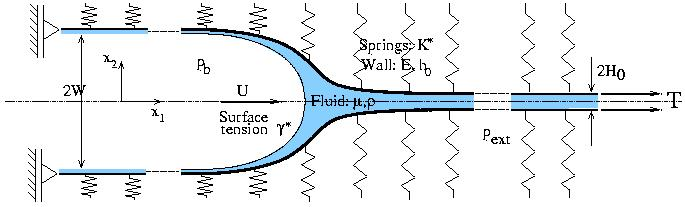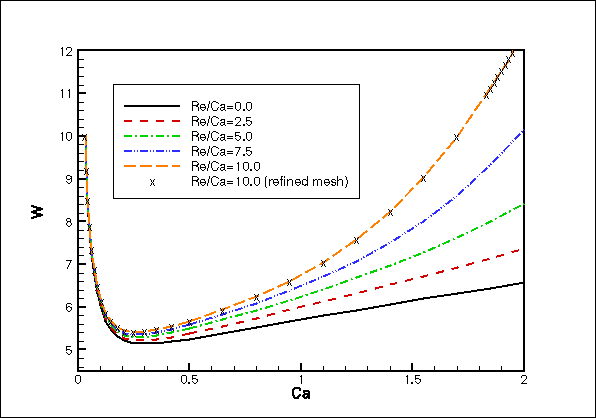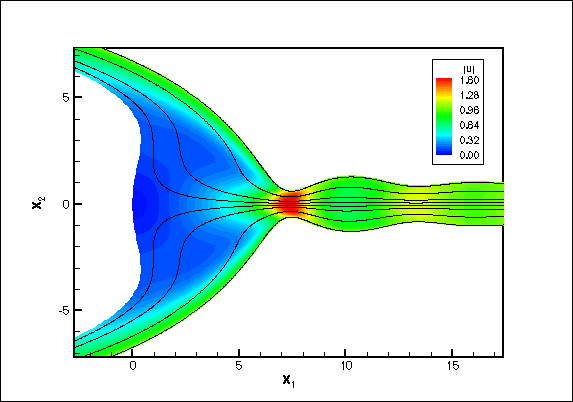# Airway Reopening: The propagation of an air finger into a collapsed elastic tube

### Background

Many pulmonary diseases result in the collapse and occlusion of parts of the lung with viscous fluid. The subsequent airway reopening is assumed to occur via the propagation of an `air finger' into the collapsed, fluid-filled part of the airway. The problem has some similarity to the scenario of the `first breath' when air has to enter the fluid-filled airways of a newborn baby for the first time. Due to the complex nature of the three-dimensional fluid-structure interaction which governs this problem (a free surface flow interacting with a strongly collapsed elastic tube), the mechanics of airway reopening are still poorly understood.

### The Mathematical Model

The figure below shows a conceptually simple mathematical model of airway reopening, first proposed by Gaver et al. (JFM 319, 1996): A positive bubble pressure pb drives an air finger into a fluid-filled 2D channel whose walls consist of thin elastic beams under large axial tension T. The walls are supported laterally on an elastic foundation which represents the parenchymal tethering.### Finite Reynolds number effects

Gaver et al. studied the problem at zero Reynolds number using a boundary element method. We re-investigated the problem, using a Finite Element solution of the fully coupled fluid-structure interaction problem. which allowed us to study the effect of fluid inertia on the system's behaviour.

#### The pressure/flow relationshipThe figure shows the channel width W, far behind the bubble tip as a function of the non-dimensional propagation speed of the bubble (expressed in terms of the capillary number Ca). Since the channel width W is proportional to the bubble pressure pb, the results show that even at these relatively modest Reynolds numbers (of about Re=10-15), fluid inertia strongly increases the bubble pressure pb required to drive the air finger at a given speed U.

#### The flow field

Fluid inertia also has a strong effect on the flow field and the corresponding wall deformation: For sufficiently high Reynolds number, the wall develops a noticeable wavy deformation pattern in the region ahead of the bubble tip. The high velocity fluid that passes through the relatively narrow `neck', which is formed by the maxima of the wavy wall displacement field, impinges on the relatively stagnant fluid ahead of the bubble tip. This can cause the air-liquid interface near the bubble tip to bulge out as shown in the figure below.The figure shows the streamlines in a frame of reference moving with the bubble tip and the colour contours represent the absolute velocities |u|. Re=15, Ca=2.0.